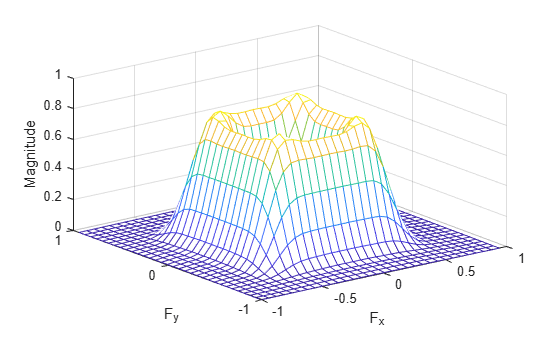freqz2

2-D frequency response

Description

example

[H,f1,f2] = freqz2(h) returns H, the 64-by-64 frequency response of h, and the frequency vectors f1 (of length 64) and f2 (of length 64). h is a two-dimensional FIR filter, in the form of a computational molecule.

freqz2 returns f1 and f2 as normalized frequencies in the range -1.0 to 1.0, where 1.0 corresponds to half the sampling frequency, or π radians.

[H,f1,f2] = freqz2(h,[n1 n2]) returns H, the n2-by-n1 frequency response of h, and the frequency vectors f1 (of length n1) and f2 (of length n2). You can also specify [n1 n2] as two separate arguments, n1,n2.

[H,f1,f2] = freqz2(h,f1, f2) returns the frequency response for the FIR filter h at frequency values in f1 and f2. These frequency values must be in the range -1.0 to 1.0, where 1.0 corresponds to half the sampling frequency, or π radians. You can also specify [f1 f2] as two separate arguments, f1, f2.

[___] = freqz2(h,___,[dx dy]) uses [dx dy] to override the intersample spacing in h. You can also specify a scalar to specify the same spacing in both the x and y dimensions.

freqz2(___) produces a mesh plot of the two-dimensional magnitude frequency response when no output arguments are specified.

Examples

collapse all

This example shows how to create a two-dimensional filter using fwind1 and how to view the filter's frequency response using freqz2.

Create an ideal frequency response.

Hd = zeros(16,16);
Hd(5:12,5:12) = 1;
Hd(7:10,7:10) = 0;

Create a 1-D window. This example uses a Bartlett window of length 16.

w = [0:2:16 16:-2:0]/16;

Create the 16-by-16 filter using fwind1 and the 1-D window. This filter gives the closest match to the ideal frequency response.

h = fwind1(Hd,w);

Display the actual frequency response of the filter.

colormap(parula(64))
freqz2(h,[32 32]);
axis ([-1 1 -1 1 0 1])Input Arguments

collapse all

2-D FIR filter, specified in the form of a computational molecule.

Data Types: single | double | int8 | int16 | int32 | int64 | uint8 | uint16 | uint32 | uint64

Number of points in the frequency response, specified as a two-element vector.

Data Types: double

Frequency vectors, specified as numeric vectors.

Data Types: double

Sample spacing, specified as a two-element vector of the form [dx dy]. The default spacing is 0.5, which corresponds to a sampling frequency of 2.0. dx determines the spacing for the x dimension and dy determines the spacing for the y dimension. If you specify a scalar, freqz2 uses the value to determine the intersample spacing in both dimensions.

Data Types: double

Output Arguments

collapse all

Frequency response, returned as a numeric array.

Frequency vector, returned as a numeric vector.

Data Types: double

Frequency vector, returned as a numeric vector.